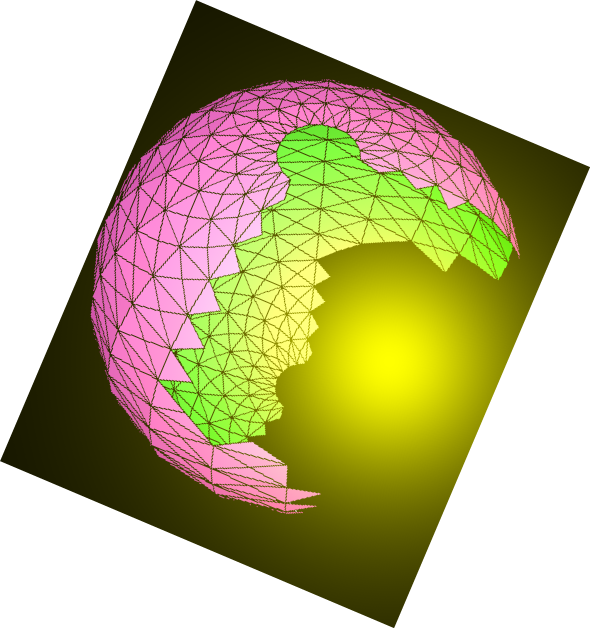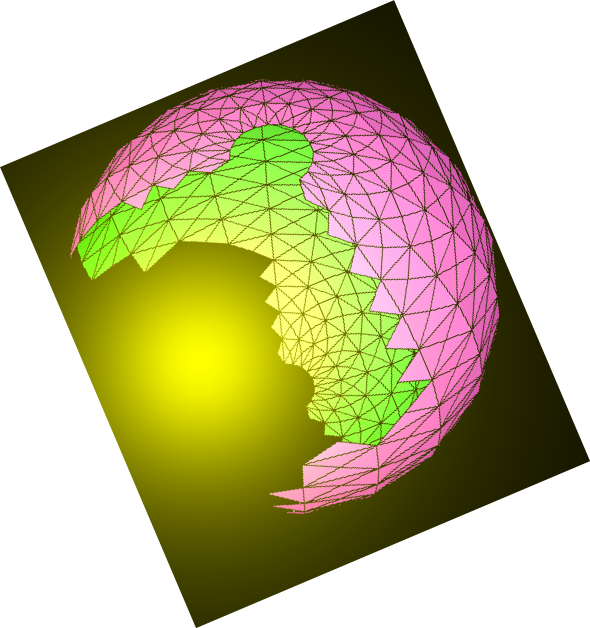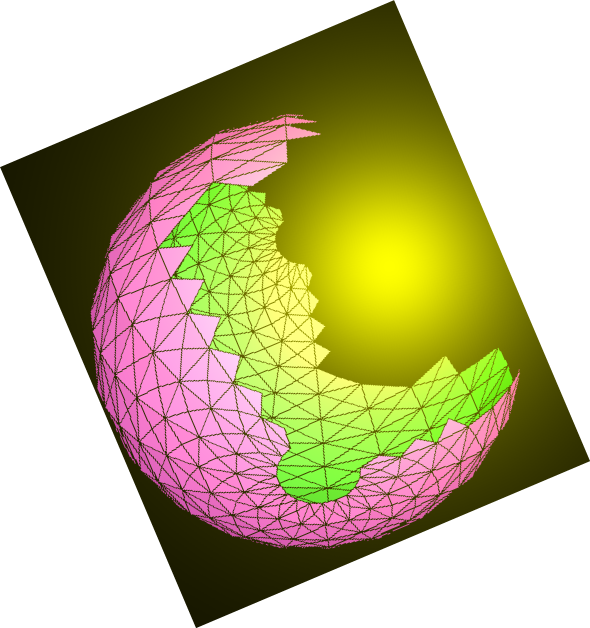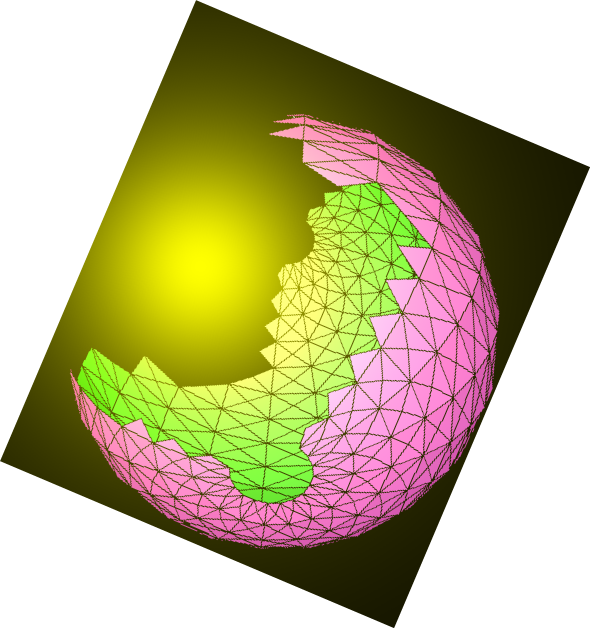﻿ JavaScript & MathScript Interactive Document Generator-Demo-06# N.S.B. Cosmic Center# JavaScript & MathScript Interactive Document Generator-Demo-06

This document is generated by N.S.B. JavaScript & MathScript Interactive Document Generator version 001.

Introduction to N.S.B. JavaScript & MathScript Interactive Document Generator

MathScript Functions

Very much like Mathematica functions, all our MathScript functions are like arrays whose elements are either numeric or alphanumeric data, as well as full-fledged functions. Unlike arrays, these functions can have heads; these can be descriptive alphanumerics or functions themselves. For example, the following is a MathScript function (remember to press the button in order to execute your MathScript input!):

###### output(1)=> F[a, b, 2.300, 5, x, y]

Here, we have given a function with six simple elements (a, b, 2.3, 5, x, y), and a head F.

In the following code sequence, we shall replace the head by by more familiar mathematical functions, and replace some elements as well. Recall from an earlier demodoc our use of the symbols %, /. and =>.

###### output(3)=> 7.300+(2*x)+(3*y)

Notice how replacing the head by the counterpart 'add' which stands for addition, the expression takes on the familiar operator form. Notice as well, that upon replacing variables in the addition expression by other existing variables, the similar terms are combined automatically.

Let go back to our original function and turn it to multiplication, and then make variable replacements:

###### output(5)=> (-11.50)*(x^2)*(y^3)

Here, you must have noticed how, in multiplication, factors are likewise combined automatically. Notice as well, that both in addition and multiplication, terms as well as factors are placed in an ordered manner.

The head of a function can be hidden. For instance, we sometimes need to use arrays and groups; these have their heads named 'arr' and 'grp', respectively. In order to convert our original function to any of these, we can of course replace the head. We can also use apply[] as follows:

###### output(7)=> (a, b, 2.300, 5, x, y)

There are many MathScript functions that can be used, in general, to manipulate other MathScript function. In the followings we shall introduce and illustrate some of them.

First, we shall begin by defining the following array--but remember that these manipulations apply to all functions whatever head they have.

###### output(8)=> {a, b, c}

The MathScript functions append[] and prepend[] can be used to add elements on the right side and on the left side respectively:

###### output(10)=> {x, a, b, c}

The functions take[] and drop[] can be used to take and drop a certain number of elements from the left and the right, depending on the sign of the integer used:

###### output(14)=> {a}

The function insert[] can be used to insert a new element at a specified location:

###### output(16)=> {a, b, c, x}

The function insertions[] gives all possible insertions:

###### output(17)=> {{x, a, b, c}, {a, x, b, c}, {a, b, x, c}, {a, b, c, x}}

Before proceeding to the next demodoc, where we shall continue to introduce more functions and features of MathScript, why don't you practice using MathScript live by clicking the program page below, and apply what you learned on this page, whichever way you like.

###### NextHome
N.S.B. Cosmic Center